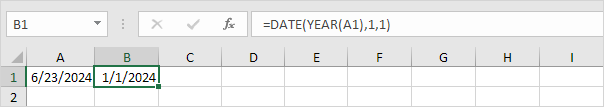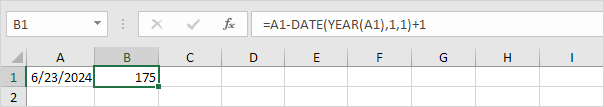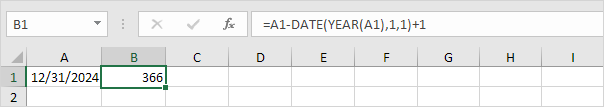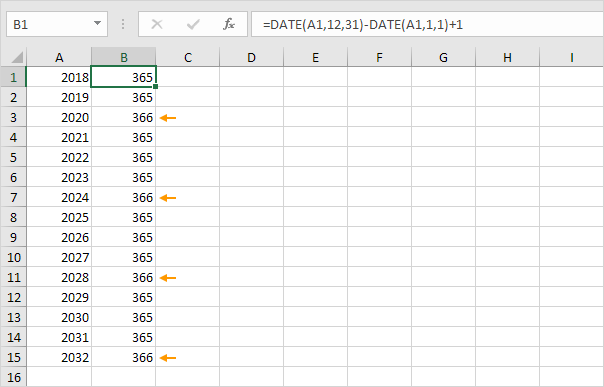# Day of the Year

An easy formula that returns the day of the year for a given date. There's no built-in function in Excel that can do this.

1. To get the year of a date, use the YEAR function.2. Use the DATE function below to return January 1, 2016. The DATE function accepts three arguments: year, month and day.3. The formula below returns the day of the year.Explanation: dates and times are stored as numbers in Excel and count the number of days since January 0, 1900. June 23, 2016 is the same as 42544. January 1, 2016 is the same as 42370. Subtracting these numbers and adding 1 gives the day of the year. 42544 - 42370 + 1 = 174 + 1 = 175.

4. 2016 is a leap year. A leap year has 366 days. December 31st is the last day of the year. We can check this.5. Bonus. The formula below calculates the number of days in a year.Conclusion: 2012, 2016, 2020 and 2024 are leap years.

Go to Next Chapter: Text Functions